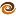# EmptyCrate

#### About Me | Contact Me | Training |ChaiScript.com | YouTube Channel

Note that some of the details on `volatile` are out of date now - 2016-03-15

(All articles in this series.)

This is the beginning of a series of articles on multithreaded programming in C++. In this first article we will look at pthreads, which are generally considered to be the “assembly language of threading.” We will start at the bottom and work our way up to higher concepts. Pthreads represent the lowest level multithreaded concept that is available on every major platform. On some platforms, pthreads are a wrapper for the operating system specific threads. On other platforms pthreads are native to the OS. First, a couple of definitions to get us started.

A path of execution separate from the main path of execution and running parallel to it. A thread provides a method by which you can have two parts of your code executing at the same time.

mutex
A method of preventing more than one thread from access a section of code at a time. If a thread has a lock on a mutex, no other thread can aquire the lock until the first thread releases its lock. Look for the functions pthread_mutex_lock and pthread_mutex_unlock in the example. Mutexes are critical for traditional multithreaded programming techniques.

The pthread library is written in C. As such, we need a C compatible way of calling the library from inside of C++. The following example represents the common way to do this:

``````class threaded_class
{
public:
: m_stoprequested(false), m_running(false)
{
}

{
}

// Create the thread and start work
// Note 1
void go()
{
assert(m_running == false);
m_running = true;
}

void stop() // Note 2
{
assert(m_running == true);
m_running = false;
m_stoprequested = true;
}

int get_fibonacci_value(int which)
{
int value = m_fibonacci_values.get(which); // Note 4
return value;
}

private:
volatile bool m_stoprequested; // Note 5
volatile bool m_running;

std::vector m_fibonacci_values;

// This is the static class function that serves as a C style function pointer
{
//All we do here is call the do_work() function
reinterpret_cast(obj)->do_work();
}

int fibonacci_number(int num)
{
switch(num)
{
case 0:
case 1:
return 1;
default:
return fibonacci_number(num-2) + fibonacci_number(num-1); // Correct 4/6/2010 based on comments
};
}

// Compute and save fibonacci numbers as fast as possible
void do_work()
{
int iteration = 0;
while (!m_stoprequested)
{
int value = fibonacci_number(iteration);
m_fibonacci_values.push_back(value);
}
}
};
``````

While this code works and is an all too common way of using threads in C++, it has several disadvantages. Note markers are made in the code and will be explained here.

### Note 1

Because we are required to pass a C style function pointer into the `pthread_create` function we end up with at least 2 (and in our case 3) functions to actually kick off the thread.

`go()` (the public interface for starting the work) calls `pthread_create()` which calls

`start_thread()` (the C style interface for starting the thread) which finally calls

`do_work()` the object level private entry into the thread.

### Note 2

`stop()` shuts down the thread and calls `pthread_join()` which does not return until the thread has been fully shutdown. What happens here if we forget to call stop? We may end up with a runaway thread that we have no way of shutting down in the best case. In the worst case we get a crash. The crash occurs when the `threaded_class` gets destroyed by the main thread of execution but the `do_work` thread is still running and is now trying to work on destructed data.

### Note 3

`pthread_mutex_lock()` is used to get an exclusive lock on the variable `m_mutex`. The locks in both `get_fibonacci_number` and `do_work` ensure that a user trying to get a fibonacci value will not try to access `m_fibonacci_values` at the same time that it is being updated by `do_work`. If an update and a read were to occur at the same time a crash is likely to happen. This is because the std::vector class resizes itself when new data is added to it.

### Note 4

What happens if `int value = m_fibonacci_values.get(which);` throws an exception? If it were to, the function `pthread_mutex_unlock` immediately below it would never get executed. If the lock is never unlocked a condition known as a deadlock occurs. When a deadlock occurs one or more threads are stopped and cannot do any work because they cannot acquire the resources they need (in our case a mutex lock). In fact, this code is susceptible to an exception being thrown if the requested fibonacci value has not yet been calculated.

### Note 5

`m_stoprequested` must be defined `volatile` for code correctness. There is a chance it would work if it were not, but without volatile it is possible to create a situation where the thread would never exit because it would not know that `m_stoprequested` had changed to `true` See the volatile article for more details.

### Note 6

If we were to forget the line `pthread_mutex_unlock(&m_mutex);` a deadlock would be created just as it would have if an exception were thrown in “Note 4.”

Coming up next, boost::threads, the “C” of multithreaded programming. Part 2 All articles in the series.

My Videos

Related